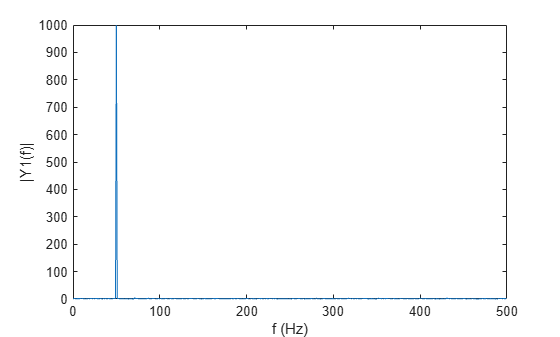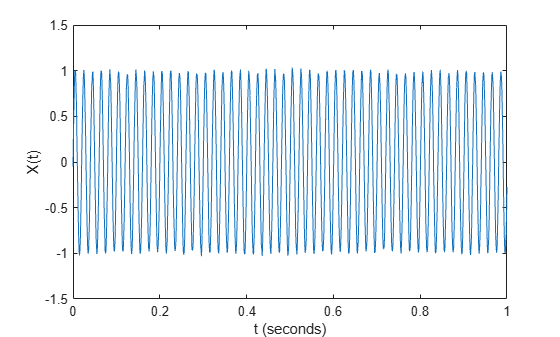# ifft

Inverse fast Fourier transform

## Syntax

``X = ifft(Y)``
``X = ifft(Y,n)``
``X = ifft(Y,n,dim)``
``X = ifft(___,symflag)``

## Description

example

````X = ifft(Y)` computes the inverse discrete Fourier transform of `Y` using a fast Fourier transform algorithm. `X` is the same size as `Y`. If `Y` is a vector, then `ifft(Y)` returns the inverse transform of the vector.If `Y` is a matrix, then `ifft(Y)` returns the inverse transform of each column of the matrix.If `Y` is a multidimensional array, then `ifft(Y)` treats the values along the first dimension whose size does not equal 1 as vectors and returns the inverse transform of each vector. ```

example

````X = ifft(Y,n)` returns the `n`-point inverse Fourier transform of `Y` by padding `Y` with trailing zeros to length `n`.```

example

````X = ifft(Y,n,dim)` returns the inverse Fourier transform along the dimension `dim`. For example, if `Y` is a matrix, then `ifft(Y,n,2)` returns the `n`-point inverse transform of each row.```

example

````X = ifft(___,symflag)` specifies the symmetry of `Y` in addition to any of the input argument combinations in previous syntaxes. For example, `ifft(Y,'symmetric')` treats `Y` as conjugate symmetric.```

## Examples

collapse all

The Fourier transform and its inverse convert between data sampled in time and space and data sampled in frequency.

Create a vector and compute its Fourier transform.

```X = [1 2 3 4 5]; Y = fft(X)```
```Y = 1×5 complex 15.0000 + 0.0000i -2.5000 + 3.4410i -2.5000 + 0.8123i -2.5000 - 0.8123i -2.5000 - 3.4410i ```

Compute the inverse transform of `Y`, which is the same as the original vector `X`.

`ifft(Y)`
```ans = 1×5 1 2 3 4 5 ```

Find the inverse Fourier transform of the single-sided spectrum that is the Fourier transform of a real signal.

Load the single-sided spectrum in the frequency domain. Show the sampling frequency and the sampling period of this spectrum.

```load Y1spectrum.mat Fs```
```Fs = 1000 ```
`T`
```T = 1.0000e-03 ```

Plot the complex magnitude of the single-sided spectrum.

```L1 = length(Y1); f = Fs/(2*L1-1)*(0:L1-1); plot(f,abs(Y1)) xlabel("f (Hz)") ylabel("|Y1(f)|")```The discrete Fourier transform of a time-domain signal has a periodic nature, where the first half of its spectrum is in positive frequencies and the second half is in negative frequencies, with the first element reserved for the zero frequency. For real signals, the discrete Fourier transform in the frequency domain is a two-sided spectrum, where the spectrum in the positive frequencies is the complex conjugate of the spectrum in the negative frequencies with half the peak amplitudes of the real signal in the time domain. To find the inverse Fourier transform of a single-sided spectrum, convert the single-sided spectrum to a two-sided spectrum.

`Y2 = [Y1(1) Y1(2:end)/2 fliplr(conj(Y1(2:end)))/2];`

Find the inverse Fourier transform of the two-sided spectrum to recover the real signal in the time domain.

`X = ifft(Y2);`

Plot the signal.

```t = (0:length(X)-1)*T; plot(t,X) xlabel("t (seconds)") ylabel("X(t)")```The `ifft` function allows you to control the size of the transform.

Create a random 3-by-5 matrix and compute the 8-point inverse Fourier transform of each row. Each row of the result has length 8.

```Y = rand(3,5); n = 8; X = ifft(Y,n,2); size(X)```
```ans = 1×2 3 8 ```

For nearly conjugate symmetric vectors, you can compute the inverse Fourier transform faster by specifying the `'symmetric'` option, which also ensures that the output is real. Nearly conjugate symmetric data can arise when computations introduce round-off error.

Create a vector `Y` that is nearly conjugate symmetric and compute its inverse Fourier transform. Then, compute the inverse transform specifying the `'symmetric'` option, which eliminates the nearly 0 imaginary parts.

`Y = [1 2:4+eps(4) 4:-1:2]`
```Y = 1×7 1.0000 2.0000 3.0000 4.0000 4.0000 3.0000 2.0000 ```
`X = ifft(Y)`
```X = 1×7 complex 2.7143 + 0.0000i -0.7213 + 0.0000i -0.0440 - 0.0000i -0.0919 + 0.0000i -0.0919 - 0.0000i -0.0440 + 0.0000i -0.7213 - 0.0000i ```
`Xsym = ifft(Y,'symmetric')`
```Xsym = 1×7 2.7143 -0.7213 -0.0440 -0.0919 -0.0919 -0.0440 -0.7213 ```

## Input Arguments

collapse all

Input array, specified as a vector, a matrix, or a multidimensional array. If `Y` is of type `single`, then `ifft` natively computes in single precision, and `X` is also of type `single`. Otherwise, `X` is returned as type `double`.

Data Types: `double` | `single` | `int8` | `int16` | `int32` | `uint8` | `uint16` | `uint32` | `logical`
Complex Number Support: Yes

Inverse transform length, specified as `[]` or a nonnegative integer scalar. Padding `Y` with zeros by specifying a transform length larger than the length of `Y` can improve the performance of `ifft`. The length is typically specified as a power of 2 or a product of small prime numbers. If `n` is less than the length of the signal, then `ifft` ignores the remaining signal values past the `n`th entry and returns the truncated result. If `n` is 0, then `ifft` returns an empty matrix.

Data Types: `double` | `single` | `int8` | `int16` | `int32` | `uint8` | `uint16` | `uint32` | `logical`

Dimension to operate along, specified as a positive integer scalar. By default, `dim` is the first array dimension whose size does not equal 1. For example, consider a matrix `Y`.

• `ifft(Y,[],1)` returns the inverse Fourier transform of each column.• `ifft(Y,[],2)` returns the inverse Fourier transform of each row.Data Types: `double` | `single` | `int8` | `int16` | `int32` | `uint8` | `uint16` | `uint32` | `logical`

Symmetry type, specified as `'nonsymmetric'` or `'symmetric'`. When `Y` is not exactly conjugate symmetric due to round-off error, `ifft(Y,'symmetric')` treats `Y` as if it were conjugate symmetric by ignoring the second half of its elements (that are in the negative frequency spectrum). For more information on conjugate symmetry, see Algorithms.

collapse all

### Discrete Fourier Transform of Vector

`Y = fft(X)` and `X = ifft(Y)` implement the Fourier transform and inverse Fourier transform, respectively. For `X` and `Y` of length `n`, these transforms are defined as follows:

`$\begin{array}{l}Y\left(k\right)=\sum _{j=1}^{n}X\left(j\right)\text{\hspace{0.17em}}{W}_{n}^{\left(j-1\right)\text{​}\left(k-1\right)}\\ X\left(j\right)=\frac{1}{n}\sum _{k=1}^{n}Y\left(k\right)\text{\hspace{0.17em}}{W}_{n}{}^{-\left(j-1\right)\text{​}\left(k-1\right)},\end{array}$`

where

`${W}_{n}={e}^{\left(-2\pi i\right)/n}$`

is one of n roots of unity.

## Algorithms

• The `ifft` function tests whether the vectors in `Y` are conjugate symmetric. If the vectors in `Y` are conjugate symmetric, then the inverse transform computation is faster and the output is real.

A function $g\left(a\right)$ is conjugate symmetric if $g\left(a\right)={g}^{*}\left(-a\right)$. However, the fast Fourier transform of a time-domain signal has one half of its spectrum in positive frequencies and the other half in negative frequencies, with the first element reserved for the zero frequency. For this reason, a vector `v` is conjugate symmetric when `v(2:end)` is equal to `conj(v(end:-1:2))`.

## Version History

Introduced before R2006a